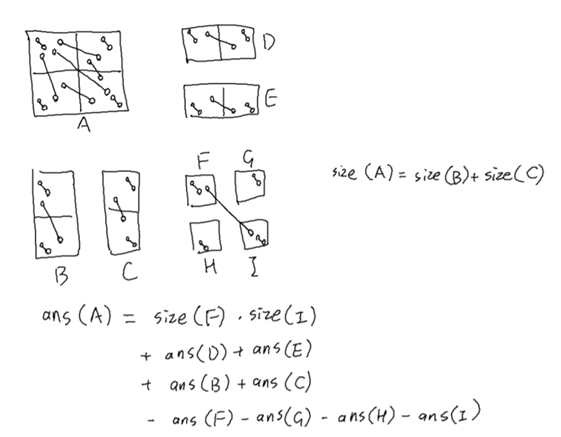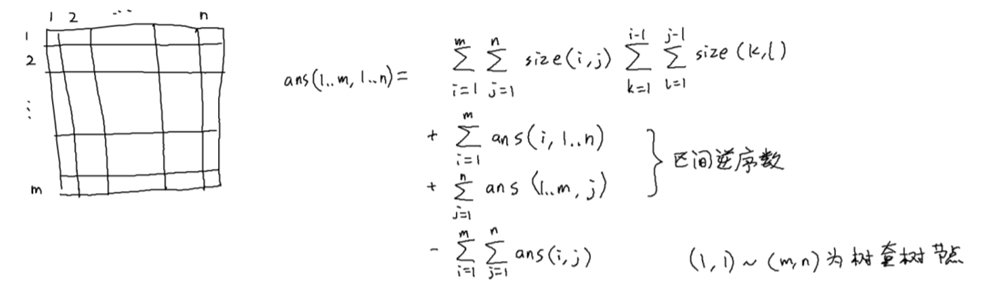# 「NOI2020」不知道咋记

（p.s.：以上是倒序）

debug 过后发现了那个没取模的地方，就 10+ byte，白给 76 分。

「补题记录」

「NOI2020」时代的眼泪$T(n,m) = \sum_{k=0}(\max_{\sum m_i = 2m} (\sum_{i=1}^{2^k} \frac{n}{2^k}\times \sqrt{m_i}))$

posted @ 2020-08-20 23:53  Tiw_Air_OAO  阅读(408)  评论(4编辑  收藏